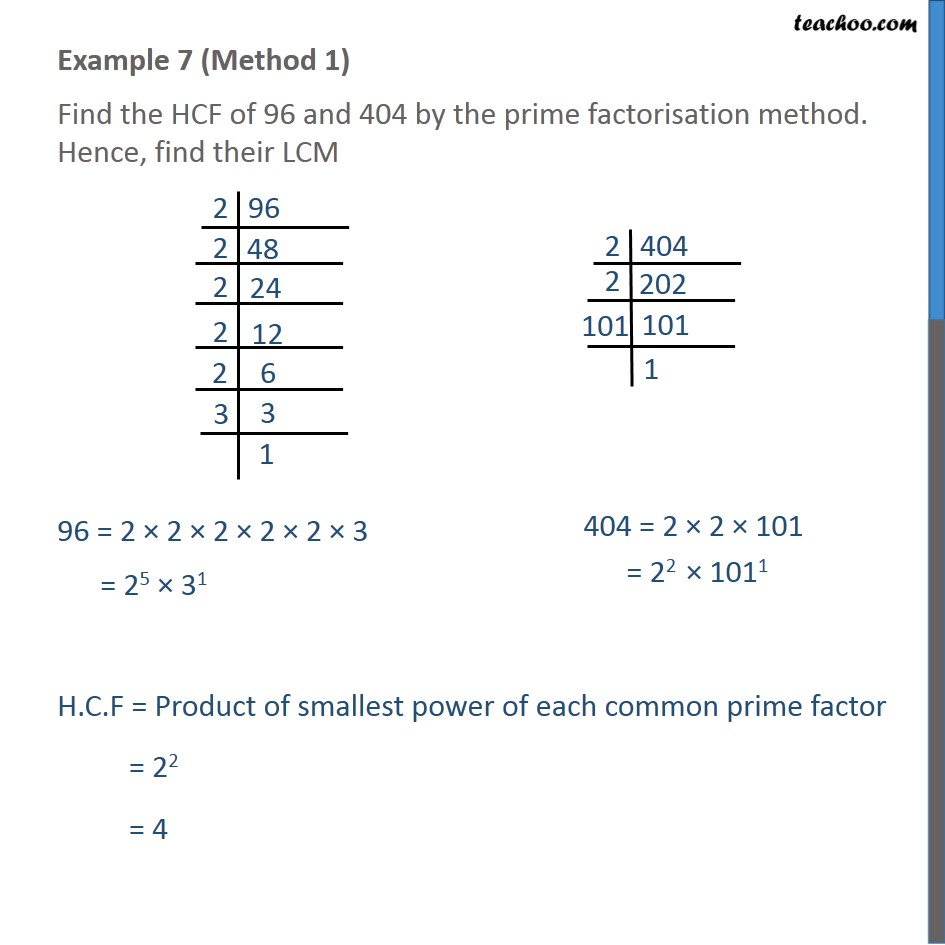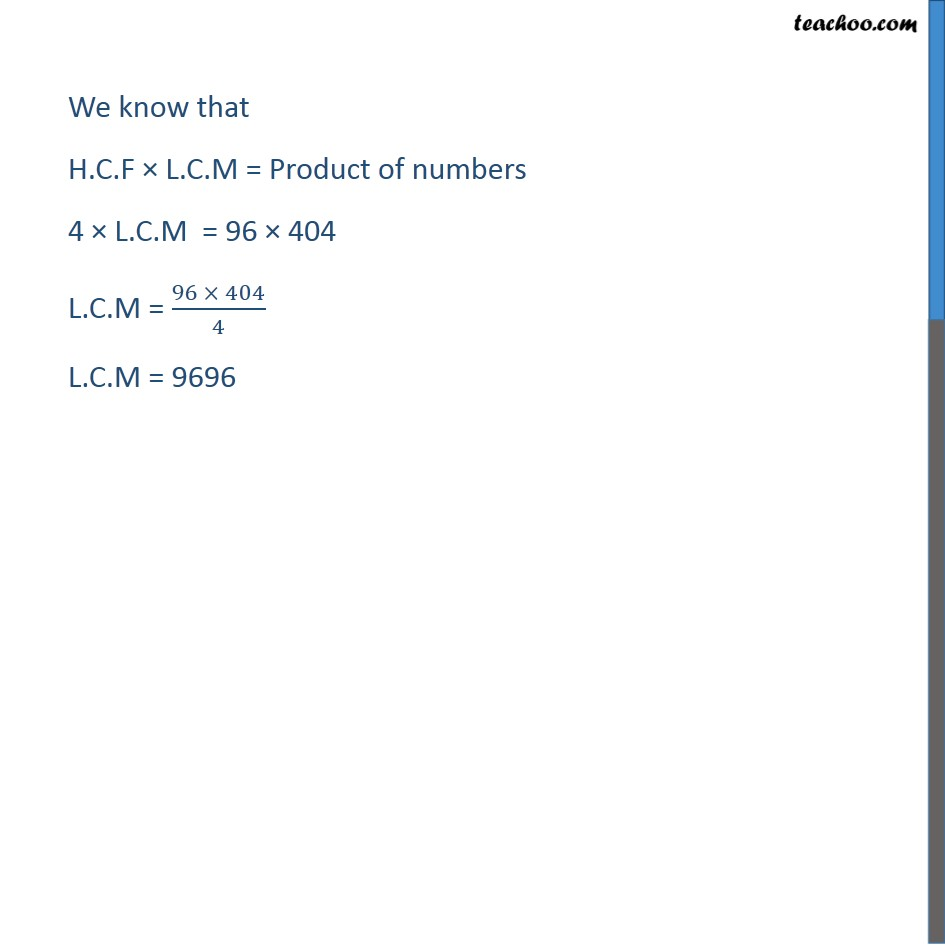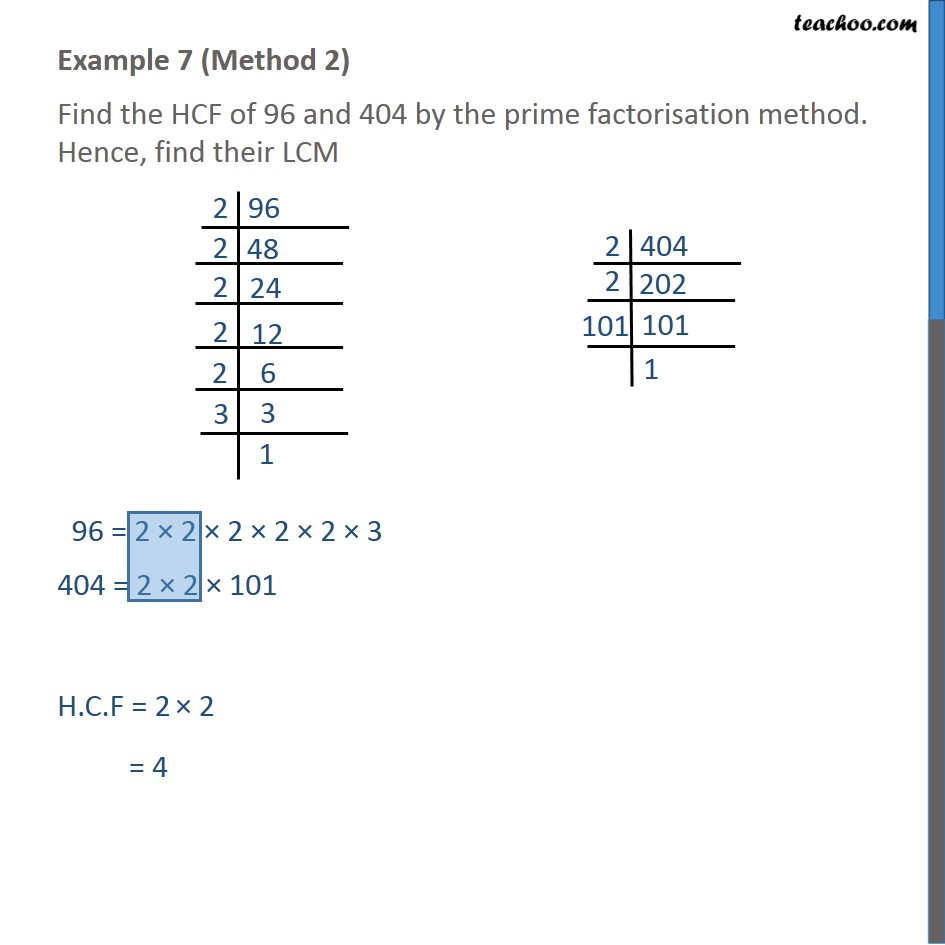Check Important Questions and Chapter Summary -https://you.tube/Real-Numbers-Class-101. Chapter 1 Class 10 Real Numbers
2. Concept wise
3. LCM/HCF

Transcript

Example 7 (Method 1) Find the HCF of 96 and 404 by the prime factorisation method. Hence, find their LCM 96 = 2 2 2 2 2 3 = 25 31 H.C.F = Product of smallest power of each common prime factor = 22 = 4 We know that H.C.F L.C.M = Product of numbers 4 L.C.M = 96 404 L.C.M = (96 404)/4 L.C.M = 9696 Example 7 (Method 2) Find the HCF of 96 and 404 by the prime factorisation method. Hence, find their LCM 96 = 2 2 2 2 2 3 404 = 2 2 101 H.C.F = 2 2 = 4 We know that H.C.F L.C.M = Product of numbers 4 L.C.M = 96 404 L.C.M = (96 404)/4 L.C.M = 9696

LCM/HCF